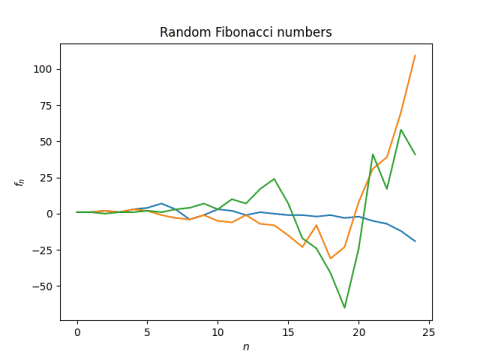# Random Fibonacci numbers

The Fibonacci numbers are defined by F1 = F2 = 1, and for n > 2,

Fn = Fn-1 + Fn-2.

A random Fibonacci sequence f is defined similarly, except the addition above is replaced with a subtraction with probability 1/2. That is, f1 = f2 = 1, and for n > 2,

fn = fn-1 + b fn-2

where b is +1 or -1, each with equal probability.

Here’s a graph a three random Fibonacci sequences.Here’s the Python code that was used to produce the sequences above.

    import numpy as np

def rand_fib(length):
f = np.ones(length)
for i in range(2, length):
b = np.random.choice((-1,1))
f[i] = f[i-1] + b*f[i-2]
return f


It’s easy to see that the nth random Fibonacci number can be as large as the nth ordinary Fibonacci number if all the signs happen to be positive. But the numbers are typically much smaller.

The nth (ordinary) Fibonacci number asymptotically approaches φn is the golden ratio, φ = (1 + √5)/2 = 1.618…

Another way to say this is thatThe nth random Fibonacci number does not have an asymptotic value—it wanders randomly between positive and negative values—but with probability 1, the absolute values satisfyThis was proved in 1960 .

Here’s a little Python code to show that we get results consistent with this result using simulation.

    N = 500
x = [abs(rand_fib(N)[-1])**(1/N) for _ in range(10)]
print(f"{np.mean(x)} ± {np.std(x)}")


This produced

1.1323 ± 0.0192

which includes the theoretical value 1.1320.

Update: The next post looks at whether every integer appear in a random Fibonacci sequence. Empirical evidence suggests the answer is yes.

## Related posts

 Furstenberg and Kesten. Products of random matrices. Ann. Math. Stat. 31, 457-469.